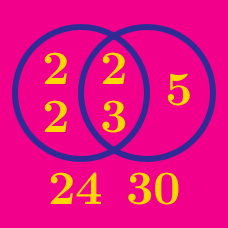Number Theory

# Relationship between GCD and LCM

What is the lowest common multiple of 7 and 11?

When the GCD of 2048 and 3072 is 1024, what is the LCM of 2048 and 3072?

$$A$$ and $$B$$ are positive integers such that

$A B=540, \quad \text{lcm}(A, B)=90.$

What is $$\gcd(A, B)?$$

If the GCD of $$A$$ and $$B$$ is $$7,$$ the LCM is $$63,$$ and $$A>B,$$ what is the value of $$B?$$

What is the lowest common multiple of 4 and 9?

×